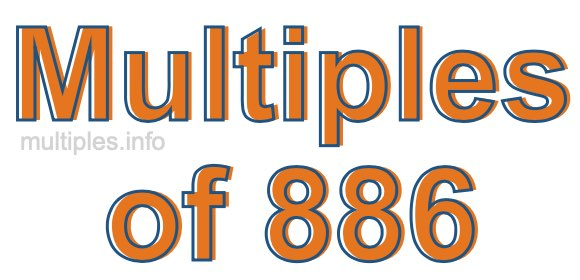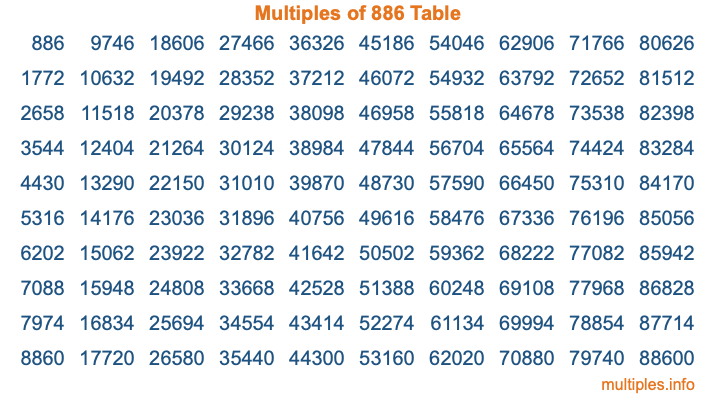Multiples of 886Welcome to the Multiples of 886 page. Here we will first teach you everything you will ever need to know about the multiples of 886, and then give you a study guide summary of everything we taught you to make sure you remember it all. Use this page to look up facts and learn information about the multiples of 886. This page will make you a multiples of eight hundred eighty-six expert!

Definition of Multiples of 886
Multiples of 886 are all the numbers that when divided by 886 equal an integer. Each of the multiples of 886 are called a multiple. A multiple of 886 is created by multiplying 886 by an integer.

Therefore, to create a list of multiples of 886, you start with 1 multiplied by 886, then 2 multiplied by 886, then 3 multiplied by 886, and so on for as long as you want. Thus, the list of the first five multiples of 886 is 886, 1772, 2658, 3544, and 4430. To see a larger list of multiples of 886, see the printable image of Multiples of 886 further down on this page. We also have a category where you can choose any nth multiple of 886.

Multiples of 886 Checker
The Multiples of 886 Checker below checks to see if any number of your choice is a multiple of 886. In other words, it checks to see if there is any number (integer) that when multiplied by 886 will equal your number. To do that, we divide your number by 886. If the the quotient is an integer, then your number is a multiple of 886.

Is  a multiple of 886?

Least Common Multiple of 886 and ...
A Least Common Multiple (LCM) is the lowest multiple that two or more numbers have in common. This is also called the smallest common multiple or lowest common multiple and is useful to know when you are adding our subtracting fractions. Enter one or more numbers below (886 is already entered) to find the LCM.

Check out our LCM Calculator if you need more details about the Least Common Multiple or if you need the LCM for different numbers for adding and subtraction fractions.

nth Multiple of 886
As we stated above, 886 is the first multiple of 886, 1772 is the second multiple of 886, 2658 is the third multiple of 886, and so on. Enter a number below to find the nth multiple of 886.

th multiple of 886

Multiples of 886 vs Factors of 886
886 is a multiple of 886 and a factor of 886, but that is where the similarities end. All postive multiples of 886 are 886 or greater than 886. All positive factors of 886 are 886 or less than 886.

Below is the beginning list of multiples of 886 and the factors of 886 so you can compare:

Multiples of 886: 886, 1772, 2658, 3544, 4430, etc.

Factors of 886: 1, 2, 443, 886

As you can see, the multiples of 886 are all the numbers that you can divide by 886 to get a whole number. The factors of 886, on the other hand, are all the whole numbers that you can multiply by another whole number to get 886.

It's also interesting to note that if a number (x) is a factor of 886, then 886 will also be a multiple of that number (x).

Multiples of 886 vs Divisors of 886
The divisors of 886 are all the integers that 886 can be divided by evenly. Below is a list of the divisors of 886.

Divisors of 886: 1, 2, 443, 886

The interesting thing to note here is that if you take any multiple of 886 and divide it by a divisor of 886, you will see that the quotient is an integer.

Multiples of 886 Table
Below is an image of the first 100 multiples of 886 in a table. The table is in chronological order, column by column. The first column has the first ten multiples of 886, the second column has the next ten multiples of 886, and so on.The Multiples of 886 Table is also referred to as the 886 Times Table or Times Table of 886. You are welcome to print out our table for your studies.

Negative Multiples of 886
Although not often discussed or needed in math, it is worth mentioning that you can make a list of negative multiples of 886 by multiplying 886 by -1, then by -2, then by -3, and so on, to get the following list of negative multiples of 886:

-886, -1772, -2658, -3544, -4430, etc.

Multiples of 886 Summary
Below is a summary of important Multiples of 886 facts that we have discussed on this page. To retain the knowledge on this page, we recommend that you read through the summary and explain to yourself or a study partner why they hold true.

There are an infinite number of multiples of 886.

A multiple of 886 divided by 886 will equal a whole number.

886 divided by a factor of 886 equals a divisor of 886.

The nth multiple of 886 is n times 886.

The largest factor of 886 is equal to the first positive multiple of 886.

886 is a multiple of every factor of 886.

886 is a multiple of 886.

A multiple of 886 divided by a divisor of 886 equals an integer.

886 divided by a divisor of 886 equals a factor of 886.

Any integer times 886 will equal a multiple of 886.

Multiples of a Number
Here you can get the multiples of another number, all with the same attention to detail as we did for multiples of 886 on this page.

Multiples of
Multiples of 887
Did you find our page about multiples of eight hundred eighty-six educational? Do you want more knowledge? Check out the multiples of the next number on our list!